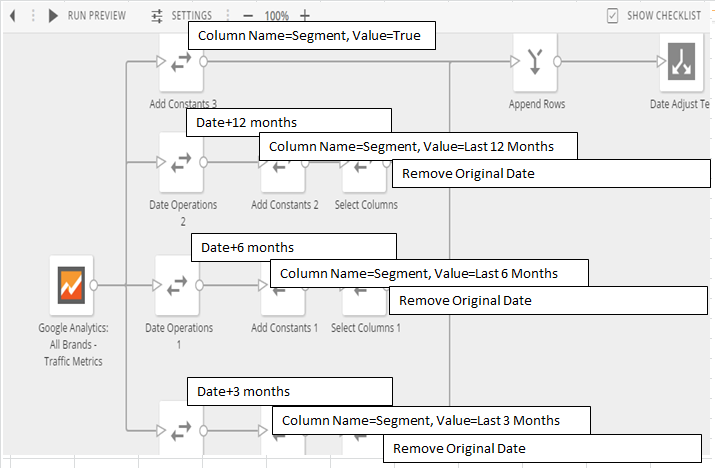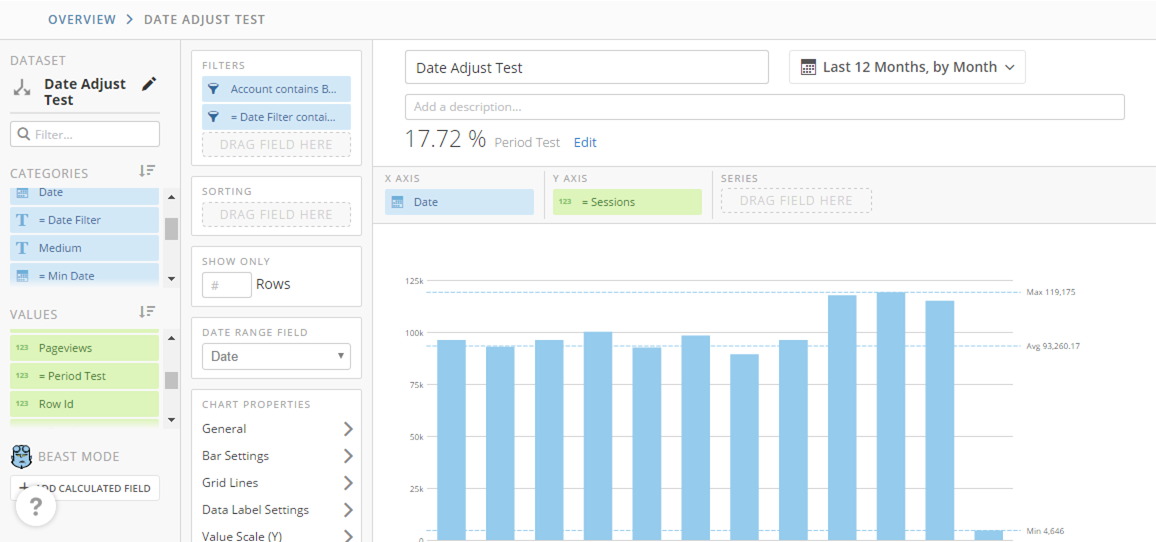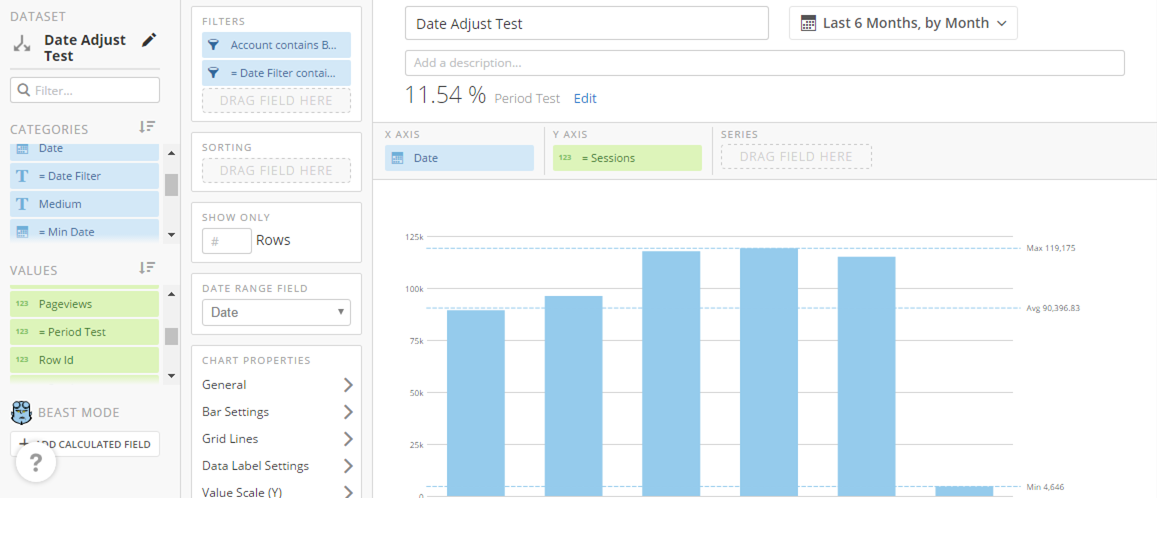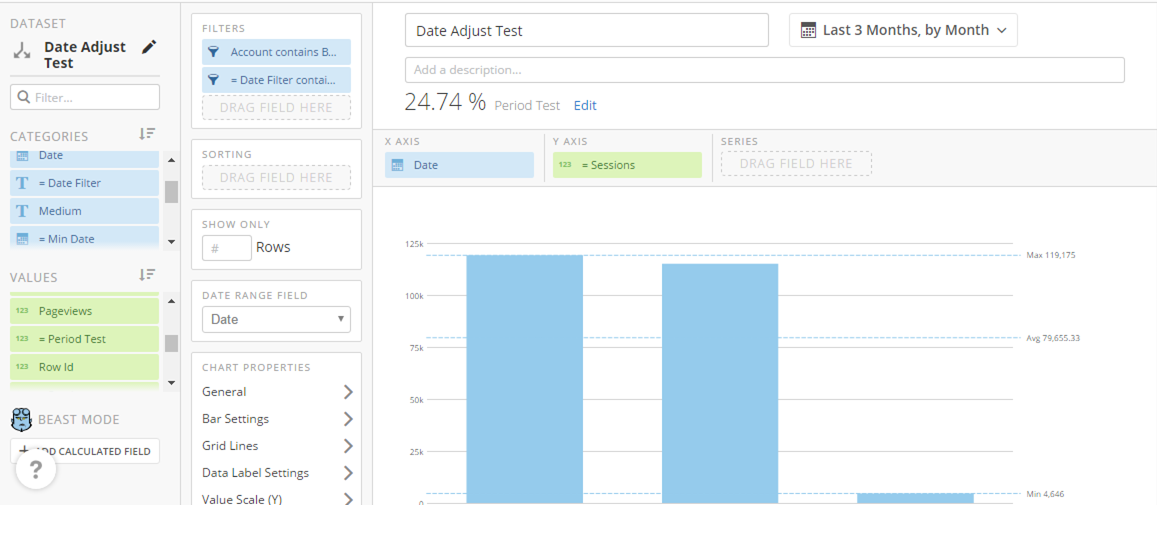# Dynamic Year over Year, Month over Month, Quarter over Quarter etc.

So we've figured out how to create YoY, MoM and QoQ calculations however we have had to rely on the date being locked to a specific time and decreasing less than 12 months back ruins the formula. I have come up with a solution to this and am posting it here to a) allow users to pick it apart and confirm if I overlooked something and b) ultimately come up with a less convoluted proces.

This is a slightly lengthy process, so please bear with me as I attempt to decribe it. If anything seems unclear, please feel free to contact me or comment.

Methodology

My method heavily relies on dataflows and essentially "tricking" the system into thinking that you are comparing the same dates against each other. Here is a snapshot of my dataflow:To summarize you are appending 3 different dateasets to the original where you have adjusted the date by a set amount of months to make it similar to the current period of timet. The beauty of this is that you can created as many segments as you want here, it just takes more time.

Formulas

In order to make the a dynamic calculation I had to use a period of time that I knew I could rely on to be constant. Personally I chose min([Date]) to get this number. This minimum date will adjust as you change the period of time and will be the basis of the dynamic period over period calculations.

First thing create a date filter as such:

(Case when `Date`< CURRENT_DATE() then 'Yes' Else 'No' End ) This will ensure that when you are comparing period over period you are only comparing the exact same time period against each other.

Next create the dynamic calculation. My calculation is below:

Case
when DATEDIFF(CURRENT_DATE(),min(`Date`)) = (DATEDIFF(CURRENT_DATE(),SUBDATE((CURRENT_DATE()-(DAYOFMONTH(CURRENT_DATE())-1)),interval 2 month))) then ((sum(case when `Segment`='True' then `Visits` else 0 end)/sum(CASE when `Segment`='Last 3 Months' then `Visits` else 0 end))-1)
when DATEDIFF(CURRENT_DATE(),min(`Date`)) = (DATEDIFF(CURRENT_DATE(),SUBDATE((CURRENT_DATE()-(DAYOFMONTH(CURRENT_DATE())-1)),interval 5 month))) then ((sum(case when `Segment`='True' then `Visits` else 0 end)/sum(CASE when `Segment`='Last 6 Months' then `Visits` else 0 end))-1)
when DATEDIFF(CURRENT_DATE(),min(`Date`)) = (DATEDIFF(CURRENT_DATE(),SUBDATE((CURRENT_DATE()-(DAYOFMONTH(CURRENT_DATE())-1)),interval 11 month))) then ((sum(case when `Segment`='True' then `Visits` else 0 end)/sum(CASE when `Segment`='Last 12 Months' then `Visits` else 0 end))-1)
End

This uses a standard (New/Old)-1 delta calculation or (New-Old)/Old and determines which segment based the date difference between the current date and the minimum date. You can then set this as the summary value to dynamically show the difference between periods. I've posted snapshots of my date below to illustrate. (Note: You need to create a new Value calculation to only sum the value when Segment=True)Again, please feel free to comment on anything I have overlooked and please upvote if this has helped you at all.

Tagged:
1